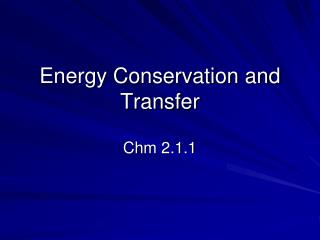DownloadDownload PresentationEnergy Conservation and Transfer

# Energy Conservation and Transfer

Télécharger la présentation## Energy Conservation and Transfer

- - - - - - - - - - - - - - - - - - - - - - - - - - - E N D - - - - - - - - - - - - - - - - - - - - - - - - - - -
##### Presentation Transcript

1. Energy Conservation and Transfer Chm 2.1.1

2. States of Matter • Solid KMT • particles packed tightly together • high attraction • Lowest energy of all states • Volume: definite • Shape: definite • Density: High (incompressible) • Types: Crystalline and Amorphous

3. States of Matter • Liquid KMT • particles are “fluid”, • less attraction • Higher energy than in solids • Volume: definite • Shape: not definite • Density: relatively high

4. States of Matter • Gases KMT • particles are spread apart • very low or no attraction • Highest energy • Volume and Shape not definite • Density: very low • able to be compressed

5. States of Matter

6. Phase Changes of Matter • Endothermic • Melting (liquefying) • Boiling • Vaporization (Evaporation) • Sublimation • Exothermic • Freezing (solidifying) • Condensing • Deposition

7. Temperature • Measures “speed” or Kinetic Energy of particles (T) • Measured in ºC or Kelvin (K) • K = ºC + 273 • Heat measures energy absorbed or released (q) • Measured in joules (J) or kilojoules (kJ)

8. Equilibrium in Changes of State • Equilibrium: a dynamic condition in which two opposing changes occur at equal rates, at the same time in a closed system

9. Equilibrium Vaporization liquid + heat energy  vapor Condensation vapor  liquid + heat energy At Equilibrium Liquid + Heat Energy ↔ Vapor

10. Vapor Pressure • Vapor Pressure = vapor molecules at equilibrium exert pressure • Effects of Temperature of Vapor Pressure • Increase Temperature = increases VP • Decrease Temperature = decrease VP • Boiling = when VP of liquid is = to the atmospheric pressure

11. Phase Diagram for H2O

12. Phase Diagram for CO2

13. Heating Curves

14. Heat Calculations • Specific heat: • The amount of energy needed to raise 1g of a substance 1°C or 1K • Cp = J/g°C or J/gK • Measured under conditions of constant pressure • All substances have their own specific heat • Cp = q/mΔT

15. Calculating Heat • Calculating Heat from Cp • q = mCpΔT Ex: Calculate the amount of heat absorbed by a 6.0g sample of aluminum when the temperature is increased from 500°C to 550°C.

16. The heating curve above gives data for water when it is cooled. How much heat energy is released from 4.0g of water when it is cooled from 100° to 0°

17. Heat of Fusion • The amount of energy required/released when melting/freezing 1g of water at 0°C • Hf = 334 J/g • q = mHf Ex: how much energy will be absorbed by a 3.0g ice cube at 0°C?

18. Heat of Vaporization • The amount of energy absorbed or released when vaporizing/condensing 1g of water at 100°C • Hv = 2,260 J/g • q = mHv Example: How much energy is absorbed when 9.36 g of water is vaporized?

19. Law of Conservation of Energy • In a closed system • Energy is cannot be created or destroyed only transferred. • heat lost in a system is gained by the surroundings • Heat gained in a system is lost by the surroundings • q lost = q gained

20. An ice cube while melting gained 25 J of energy. How much energy was lost by its surroundings? • A hot piece of metal is put into a cup of water. What will happen to the metal? What will happen to the water? • Copper was heated and placed in a cup of water at room temperature (25°C). The water’s temperature rose 30°C. How much energy did the copper loose?